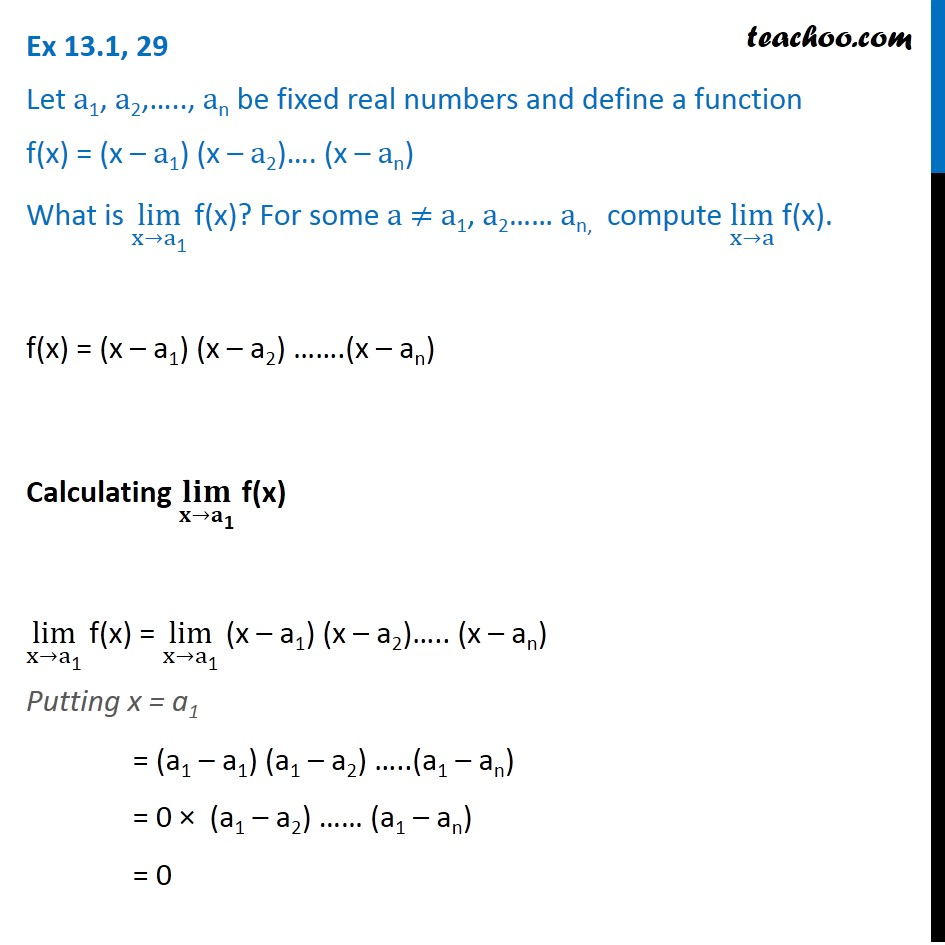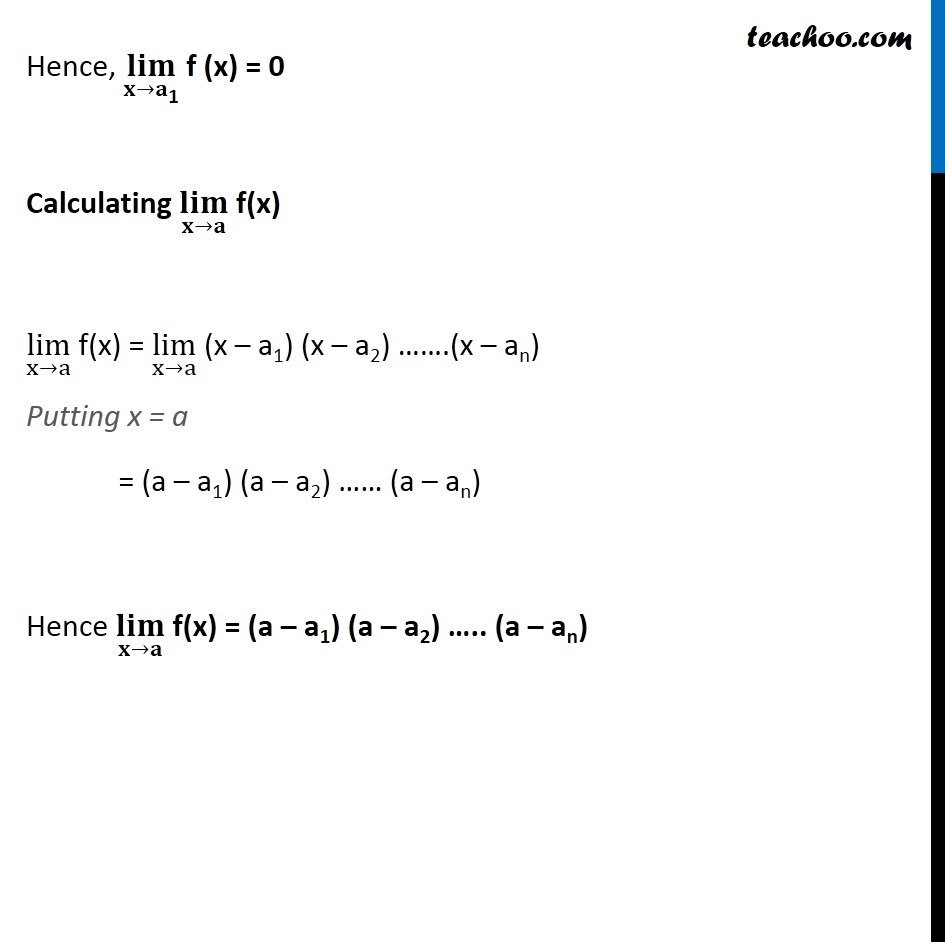Subscribe to our Youtube Channel - https://you.tube/teachoo

1. Chapter 13 Class 11 Limits and Derivatives
2. Serial order wise
3. Ex 13.1

Transcript

Ex 13.1, 29 Let a1, a2,….., an be fixed real numbers and define a function f(x) = (x – a1) (x – a2)…. (x – an) What is lim┬(x→a"1" ) f(x)? For some a ≠ a1, a2…… an, compute lim┬(x→a) f(x). f(x) = (x – a1) (x – a2) …….(x – an) Calculating (𝐥𝐢𝐦)┬(𝐱→𝐚"1" ) f(x) lim┬(x→a"1" ) f(x) = lim┬(x→a"1" ) (x – a1) (x – a2)….. (x – an) Putting x = a1 = (a1 – a1) (a1 – a2) …..(a1 – an) = 0 × (a1 – a2) …… (a1 – an) = 0 Hence, (𝐥𝐢𝐦)┬(𝐱→𝐚"1" ) f (x) = 0 Calculating (𝐥𝐢𝐦)┬(𝐱→𝐚) f(x) lim┬(x→a) f(x) = lim┬(x→a) (x – a1) (x – a2) …….(x – an) Putting x = a = (a – a1) (a – a2) …… (a – an) Hence (𝐥𝐢𝐦)┬(𝐱→𝐚) f(x) = (a – a1) (a – a2) ….. (a – an)

Ex 13.1